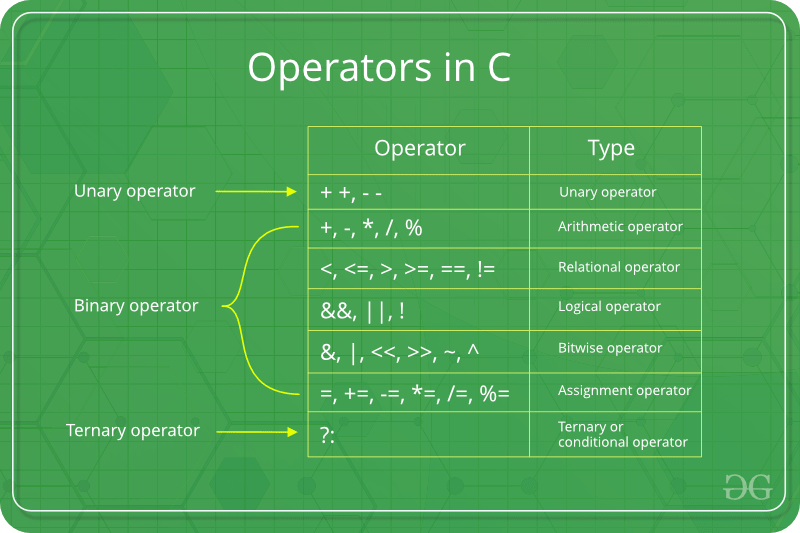# operators in c?## OPRATORS IN C  : -

Operators are the foundation of any programming language. Thus the functionality of C  programming language is incomplete without the use of operators. We can define operators as symbols that help us to perform specific mathematical and logical computations on operands. In other words, we can say that an operator operates the operands.### For example : -

consider the below statement:

c = a + b;

Here, ‘+’ is the operator known asaddition operator and ‘a’ and ‘b’ are operands. The addition operator tells the compiler to add both of the operands ‘a’ and ‘b’.

C/C++ has many built-in operator types and they are classified as follows:

### Arithmetic Operators :

These are the operators used to perform arithmetic/mathematical operations on operands. Examples: (+, -, *, /, %,++,–). Arithmetic operator are of two types:Unary Operators: Operators that operates or works with a single operand are unary operators. For example: (++ , –)Binary Operators: Operators that operates or works with two operands are binary operators. For example: (+ , – , * , /) To learn Arithmetic Operators in details visit this link.### Relational Operators :

These are used for comparison of the values of two operands. For example, checking if one operand is equal to the other operand or not, an operand is greater than the other operand or not etc. Some of the relational operators are (==, >= , <= ).### Logical Operators:

Logical Operators are used to combine two or more conditions/constraints or to complement the evaluation of the original condition in consideration. The result of the operation of a logical operator is a boolean value either true or false. For example, the logical ANDrepresented as ‘&&’ operator in C or C++ returns true when both the conditions under consideration are satisfied. Otherwise it returns false. Therfore, a && b returns true when both a and b are true (i.e. non-zero). To learn about different logical operators in details please visit this link.### Bitwise Operators:

The Bitwise operators is used to perform bit-level operations on the operands. The operators are first converted to bit-level and then the calculation is performed on the operands. The mathematical operations such as addition, subtraction, multiplication etc. can be performed at bit-level for faster processing. For example, thebitwise AND represented as & operator in C or C++ takes two numbers as operands and does AND on every bit of two numbers. The result of AND is 1 only if both bits are 1. To learn bitwise operators in details, visit this link.### Assignment Operators:

Assignment operators are used to assign value to a variable. The left side operand of the assignment operator is a variable and right side operand of the assignment operator is a value. The value on the right side must be of the same data-type of variable on the left side otherwise the compiler will raise an error.Different types of assignment operators are shown below:

1.  =

This is the simplest assignment operator. This operator is used to assign the value on the right to the variable on the left.

For example:

a = 10; b = 20; ch = 'y';

2. +=

This operator is combination of ‘+’ and ‘=’ operators. This operator first adds the current value of the variable on left to the value on right and then assigns the result to the variable on the left.

Example:(a += b) can be written as (a = a + b)

If initially value stored in a is 5. Then (a += 6) = 11.

3 . -=

This operator is combination of ‘-‘ and ‘=’ operators. This operator first subtracts the value on right from the current value of the variable on left and then assigns the result to the variable on the left.

Example:(a -= b) can be written as (a = a - b)

If initially value stored in a is 8. Then (a -= 6) = 2.

4. *=

This operator is combination of ‘*’ and ‘=’ operators. This operator first multiplies the current value of the variable on left to the value on right and then assigns the result to the variable on the left.

Example:(a *= b) can be written as (a = a * b)

If initially value stored in a is 5. Then (a *= 6) = 30.

5 .  /=

This operator is combination of ‘/’ and ‘=’ operators. This operator first divides the current value of the variable on left by the value on right and then assigns the result to the variable on the left.

Example:(a /= b) can be written as (a = a / b)

If initially value stored in a is 6. Then (a /= 2) = 3.